# Voltage Dividers

Next Page: Transistor Circuits

## What is a voltage divider?

A voltage divider consists of two resistances R1 and R2 connected in series across a supply voltage Vs. The supply voltage is divided between the two resistances to give an output voltage Vo which is the voltage across R2.

An important use of voltage dividers is to connect input transducers to circuits.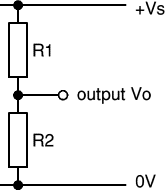The output voltage Vo depends on the size of R2 relative to R1:

• If R2 is much smaller than R1, Vo is small (low, almost 0V) because most of the voltage is across R1.
• If R2 is about the same as R1, Vo is about half Vs because the voltage is shared about equally between R1 and R2.
• If R2 is much larger than R1, Vo is large (high, almost Vs) because most of the voltage is across R2.

If you need a precise value for the output voltage Vo you can use this formula:

 Voltage divider output, Vo = Vs × R2 R1 + R2

Important: this formula and the rough rules given above assume that negligible current flows from the output. This is true if Vo is connected to a device with a high resistance such as voltmeter or an IC input. For further information please see the page on impedance. If the output is connected to a transistor Vo cannot become much greater than 0.7V because the transistor's base-emitter junction behaves like a diode.

#### Potential dividers

Voltage dividers are also known as potential dividers, a name which comes from potential difference (the proper name for voltage).

## Using an input transducer (sensor) in a voltage divider

Most input transducers (sensors) vary their resistance and usually a voltage divider is used to convert this to a varying voltage which is more useful. The voltage signal can be fed to other parts of the circuit, such as the input to an IC or a transistor switch.

The sensor is one of the resistances in the voltage divider. It can be at the top (near +Vs) or at the bottom (near 0V), the choice is determined by when you want a large value for the output voltage Vo:

• Put the sensor at the top (near +Vs) if you want a large Vo when the sensor has a small resistance.
• Put the sensor at the bottom (near 0V) if you want a large Vo when the sensor has a large resistance.

Next you need to choose a value for the resistor (R) which makes up the voltage divider.

## Choosing a resistor value

The value of the resistor R determines the range (maximum and minimum values) of the output voltage Vo. For best results you need Vo to have a large range and this is achieved if R is much larger than the sensor's minimum resistance but much smaller than its maximum resistance.

Use a multimeter to find the minimum and maximum values of the sensor's resistance, there is no need to be precise - approximate values will do. Then use the formula to choose a value for resistor R:

 R = square root of (Rmin × Rmax)

Rmin = sensor's minimum resistance
Rmax = sensor's maximum resistance

Choose a standard value for R which is close to the calculated value.

For example if your LDR has Rmin = 100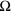and Rmax = 1M: R = square root of (100 × 1M) = 10k.

### Swapping the resistor and sensor

The resistor and sensor can be swapped over to invert the action of the voltage divider. For example an LDR has a high resistance when dark and a low resistance when brightly lit:

• LDR at top (near +Vs) makes Vo high in bright light.
• LDR at bottom (near 0V) makes Vo high in the dark.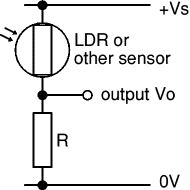Vo high in bright light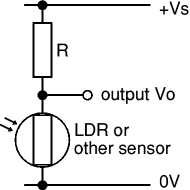Vo high in dark

## Using a variable resistor

A variable resistor may be used in place of the fixed resistor R. It will enable you to adjust the output voltage Vo for a given resistance of the sensor. For example you can use a variable resistor to set the exact brightness level which makes an IC change state.

The variable resistor value should be larger than the fixed resistor value it replaces. For finer control you can use a fixed resistor in series with the variable resistor. For example if a 10kfixed resistor is suitable you could replace it with a fixed 4.7kresistor in series with a 10kvariable resistor, allowing you to adjust the resistance from 4.7k to 14.7k.

#### Take care using variable resistors with transistors

If you are planning to use a variable resistor connected between the +Vs supply and the base of a transistor you must include a fixed resistor in series with the variable resistor. This is to prevent excessive base current destroying the transistor when the variable resistor is reduced to zero. For further details see the Transistor Circuits page.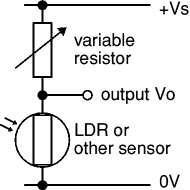The sensor and variable
resistor can be swapped
over if necessary

Next Page: Transistor Circuits | Study